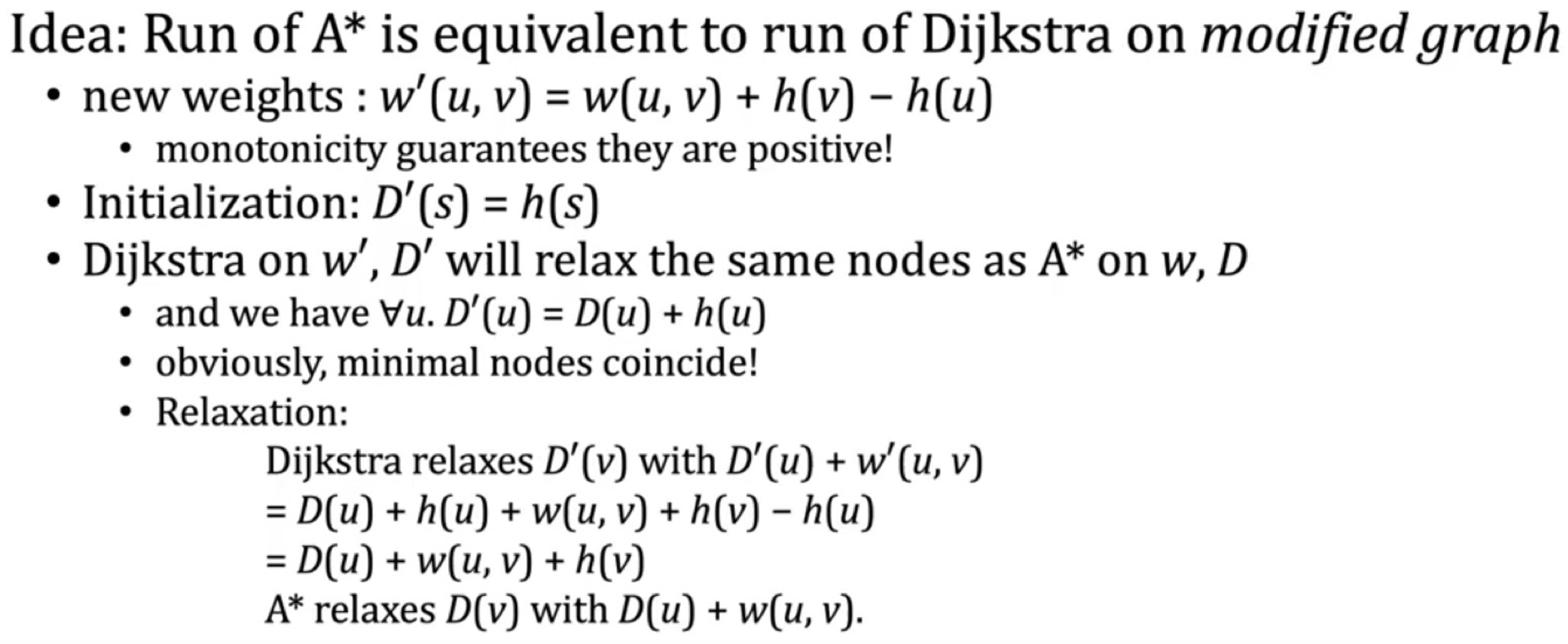# 数据结构与算法： Dijkstra与A*

## Heuristics：Dijkstra and A*

Posted by R1NG on February 25, 2022 Viewed Times

# 启发式图算法: Dijkstra 和 A*

## 1. Dijkstra 算法

### 1. 原理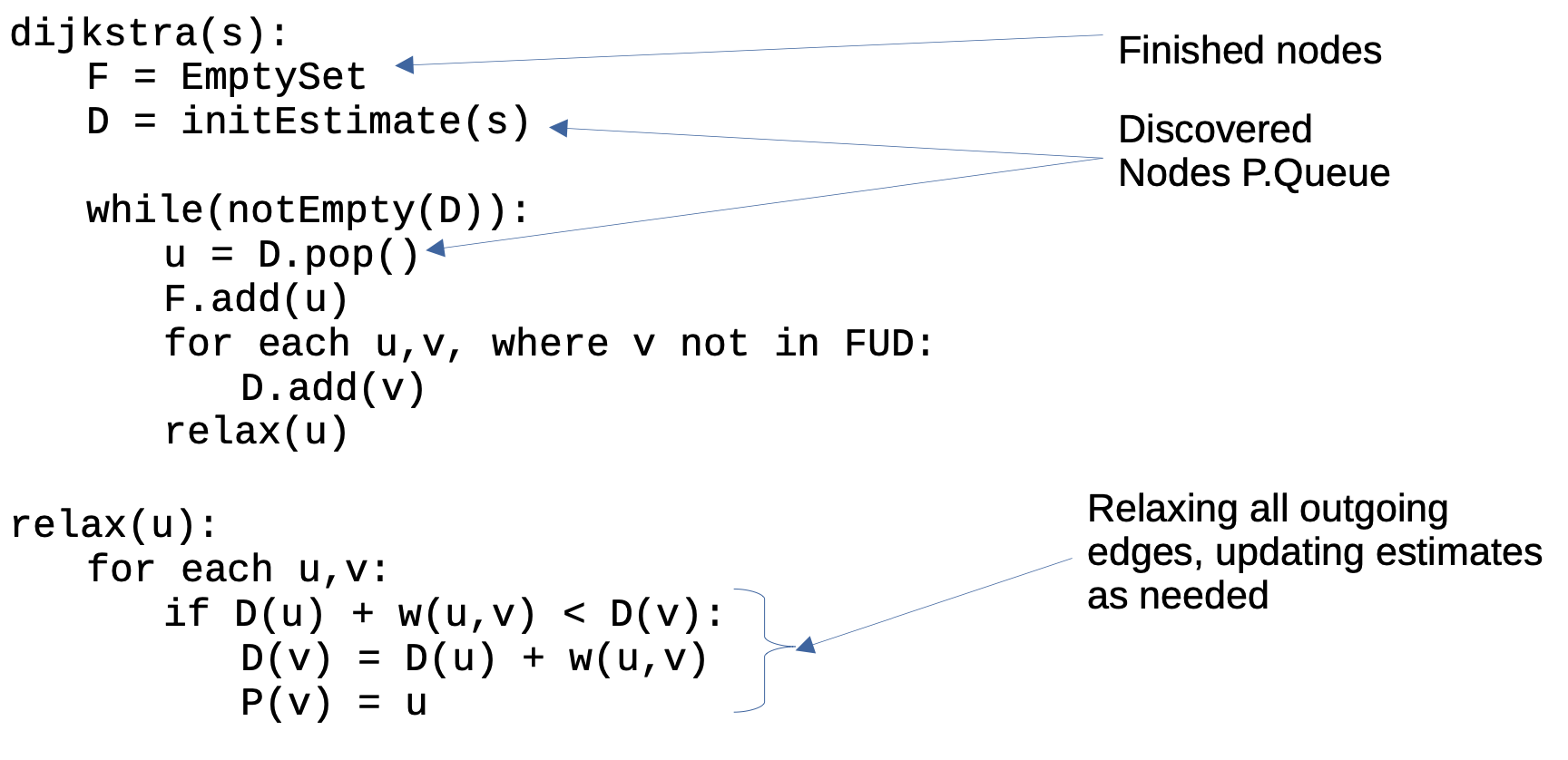Dijkstra 算法利用了 “单源最短路径的子路径仍然是最短路径” 的性质, 使用贪心的思想逐步构建局部最优的最短子路径: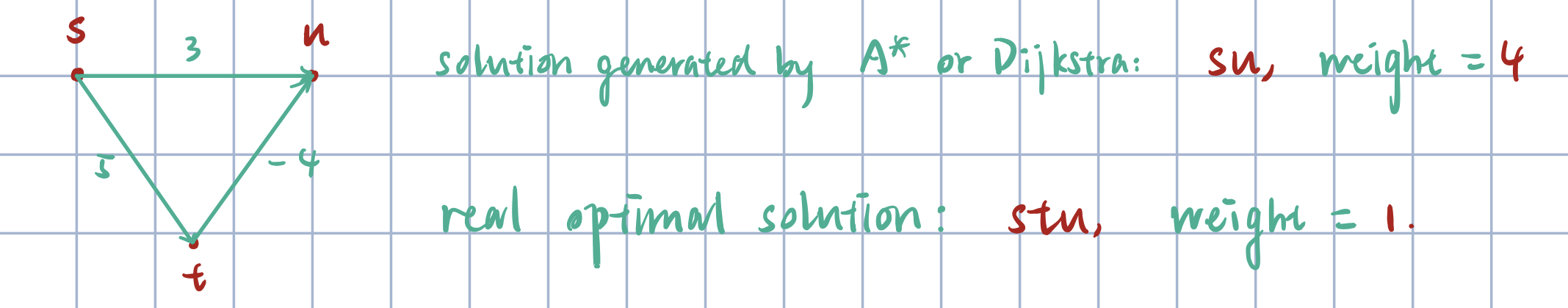同时注意, 在这里 Dijkstra 算法的 initEstimate() 逻辑和 Bellman-Ford 算法是 一致的: 只有起始节点的目标路径权重初始估计为 $0$, 其余任何节点的估计都是 $\infty$.

### 2. 实现

Dijkstra 算法需要一个优先队列用于存储已发现但待检查的点. 由于我们关心路径权重估计更小的点, 因此在此处一般使用 最小堆 实现优先队列.

### 3. 性质

1. 每条边最多被松弛一次.
2. 任何节点最多被插入进优先队列一次, 同样地也只会被从中删除一次, 没有多余操作.

$O((\vert E \vert + \vert V \vert) \cdot \log(\vert V \vert)).$

## 2. A* 算法

### 1. 原理

Dijkstra 算法仍然存在的问题是: 虽然它无需再如 Bellman-Ford 算法一般, 不用再去考虑任何不可达点, 但它仍然需要对所有的可达点遍历一遍才能得出结果. A* 算法在其基础上使用启发式方法对搜索空间进一步进行剪枝, 从而获得了更高的执行效率.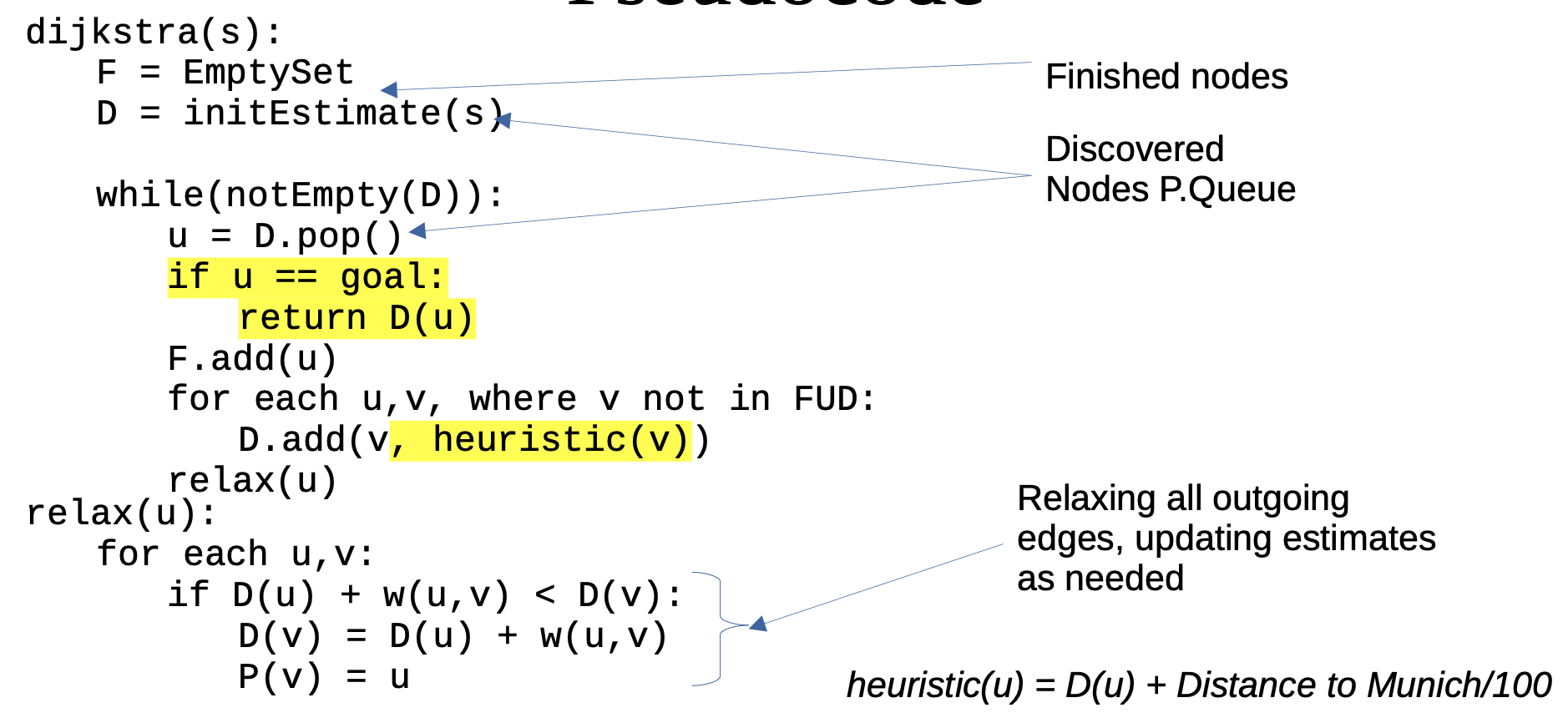### 2. 差异

A* 算法的启发式与 Dijkstra 的差异在:

1. 后者完全基于 权重 评价生成的部分解的优劣, 前者的评价标准综合了 部分解当前的尾节点到起始节点的距离 (也就是权重) 与 尾节点到目标节点的距离, 从而具有指向性: 权重越小, 距离目标节点越近的部分解越有可能被选定从而构造完整解.

$\text{heuristic(u)} = D(u) + \text{distance}(u, \text{target\_node})$
2. 由于对可选部分解排序方式的转变, 最终完整解一旦在某一步中进入我们的选择范围内, 就一定会因为启发值最小而被优先选择, 从而剩下的所有较差解都不会再被考虑, 达到了剪枝的效果 (如 Lab5 中优化的 BNB).

### 3. 启发性

$h(u) \leqslant \delta(u, t)$

$h(a) \leqslant w(a, b) + h(b).$

## 3. Dijkstra 和 A* 算法的正确性

### 1. Dijkstra 算法的正确性

Dijkstra 算法的不变量是:

1. 对于任何位于 ”已完成检查集合“ $F$ 中的点 $u$, 从起始节点到该点最短路径的权重估计一定都是精确的:

$D(u) = \delta(u).$
2. 对任何在某个位于 $F$ 中的点 $u$ 之后插入原来的路径的点 $v$, 从起始节点到 $v$ 的最短路径的权重估计 $D(v)$ 有下列的上界:

$\forall v, ~ D(v) \leqslant \delta(u) + w(u, v).$
3. 对起始节点到边集 $V$ 中任何点的最短路径的权重估计都是 过高的估计:

$\forall u \in V. ~ D(u) \geqslant \delta(u).$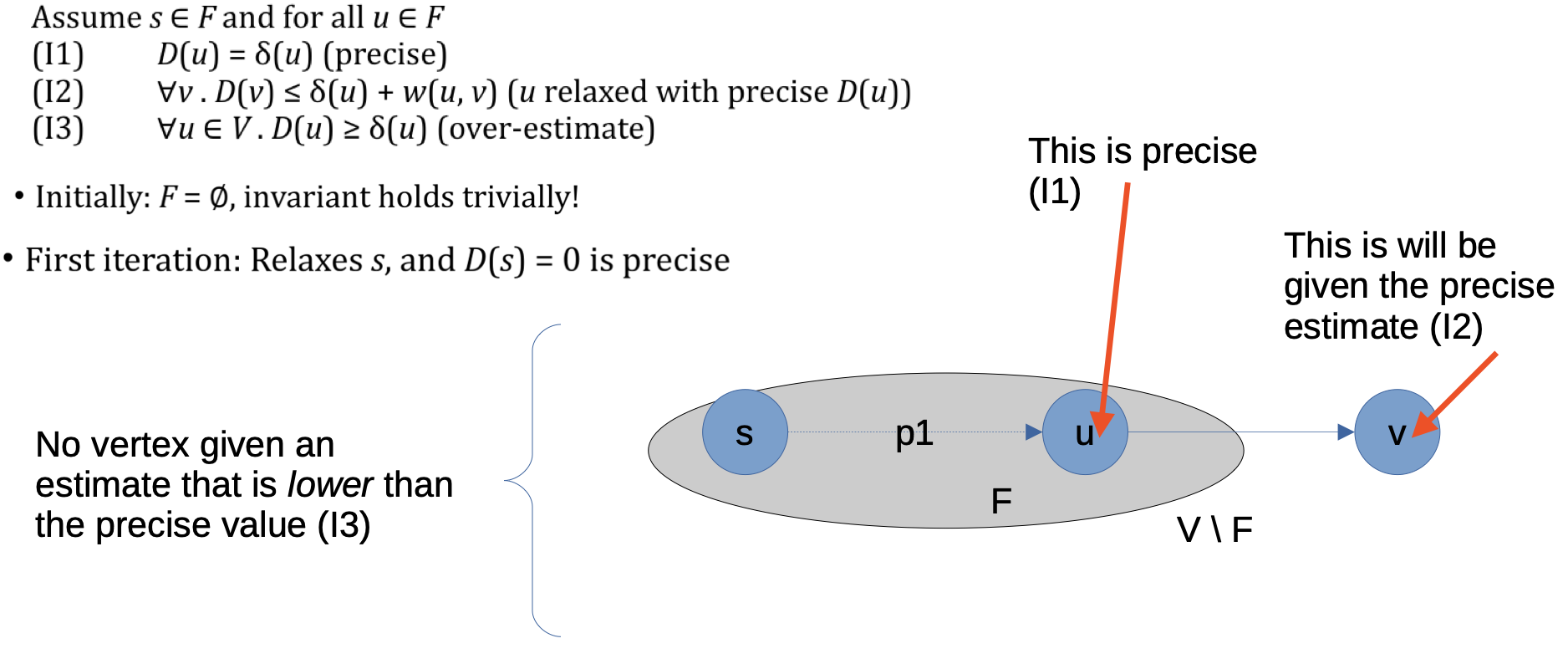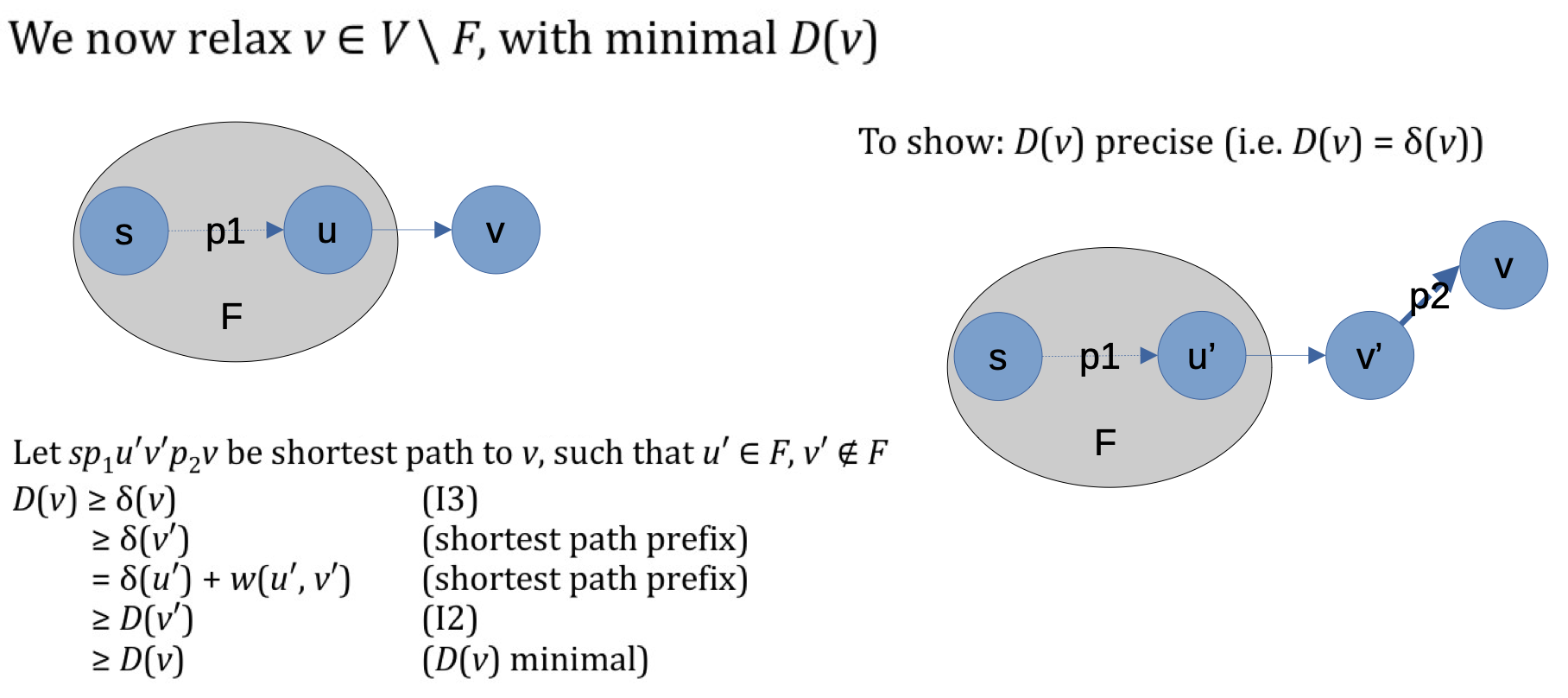### 2. A* 算法的正确性